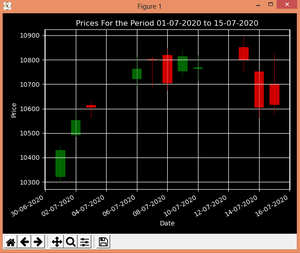# Plot Candlestick Chart using mplfinance module in Python

Candlestick chart are also known as a Japanese chart. These are widely used for technical analysis in trading as they visualize the price size within a period. They have four points Open, High, Low, Close (OHLC). Candlestick charts can be created in python using a matplotlib module called mplfinance

#### Installation:

`pip install mplfinance`

## mplfinance.candlestick_ohlc()

This function is used to plot Candlestick charts.

Syntax: mplfinance.candlestick_ohlc(ax, quotes, width=0.2, colorup=’k’, colordown=’r’, alpha=1.0)
Parameters:

• ax: An Axes instance to plot to.
• quotes: Aequence of (time, open, high, low, close, …) sequences.
• width: Fraction of a day for the rectangle width.
• colorup: The color of the rectangle where close >= open.
• colordown: The color of the rectangle where close < open.
• alpha: (float) The rectangle alpha level.

Returns: returns (lines, patches) where lines are a list of lines added and patches is a list of the rectangle patches added.

To plot the chart, we will take data from NSE for the period 01-07-2020 to 15-07-2020, the data is available for download in a csv file, or can be downloaded from here. For this example, it is saved as ‘data.csv’.
We will use the pandas library to extract the data for plotting from data.csv.

Below is the implementation:

## Python3

 `# import required packages ` `import` `matplotlib.pyplot as plt ` `from` `mplfinance ``import` `candlestick_ohlc ` `import` `pandas as pd ` `import` `matplotlib.dates as mpdates ` ` `  `plt.style.use(``'dark_background'``) ` ` `  `# extracting Data for plotting ` `df ``=` `pd.read_csv(``'data.csv'``) ` `df ``=` `df[[``'Date'``, ``'Open'``, ``'High'``,  ` `         ``'Low'``, ``'Close'``]] ` ` `  `# convert into datetime object ` `df[``'Date'``] ``=` `pd.to_datetime(df[``'Date'``]) ` ` `  `# apply map function ` `df[``'Date'``] ``=` `df[``'Date'``].``map``(mpdates.date2num) ` ` `  `# creating Subplots ` `fig, ax ``=` `plt.subplots() ` ` `  `# plotting the data ` `candlestick_ohlc(ax, df.values, width ``=` `0.6``, ` `                 ``colorup ``=` `'green'``, colordown ``=` `'red'``,  ` `                 ``alpha ``=` `0.8``) ` ` `  `# allow grid ` `ax.grid(``True``) ` ` `  `# Setting labels  ` `ax.set_xlabel(``'Date'``) ` `ax.set_ylabel(``'Price'``) ` ` `  `# setting title ` `plt.title(``'Prices For the Period 01-07-2020 to 15-07-2020'``) ` ` `  `# Formatting Date ` `date_format ``=` `mpdates.DateFormatter(``'%d-%m-%Y'``) ` `ax.xaxis.set_major_formatter(date_format) ` `fig.autofmt_xdate() ` ` `  `fig.tight_layout() ` ` `  `# show the plot ` `plt.show()`

Output :Candlestick Chart

Attention geek! Strengthen your foundations with the Python Programming Foundation Course and learn the basics.

To begin with, your interview preparations Enhance your Data Structures concepts with the Python DS Course.

My Personal Notes arrow_drop_upCheck out this Author's contributed articles.

If you like GeeksforGeeks and would like to contribute, you can also write an article using contribute.geeksforgeeks.org or mail your article to contribute@geeksforgeeks.org. See your article appearing on the GeeksforGeeks main page and help other Geeks.

Please Improve this article if you find anything incorrect by clicking on the "Improve Article" button below.

Article Tags :

Be the First to upvote.

Please write to us at contribute@geeksforgeeks.org to report any issue with the above content.